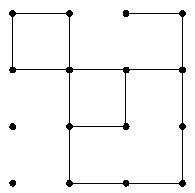Online JudgeProblem SetAuthorsOnline ContestsUser
Web Board
F.A.Qs
Statistical Charts
Problems
Submit Problem
Online Status
Prob.ID:
Register
Authors ranklist
Current Contest
Past Contests
Scheduled Contests
Award Contest
Register
Language:
Squares
 Time Limit: 15000MS Memory Limit: 131072K Total Submissions: 1144 Accepted: 343

Description

A children's board game consists of a square array of dots that contains lines connecting some dots. One part of the game requires that the players count the number of squares that are formed by these lines. For example, in the figure shown below, there are 3 squares, 2 of size 1 and 1 of size 2 , so the total number of squares is 3. (The "size" of a square is the number of lines segments required to form a side.)Your task is to write a program to count the number of all the possible squares.

Input

The input represents a series of game boards. Each board consists of a description of a square array of n ^ 2 dots (where 2 <= n <= 1500) and some interconnecting horizontal and vertical lines. A record of a single board with n ^ 2 dots and m (m <= 300000 )interconnecting lines is formatted as follows:

Line 1: "n" the number of dots in a single row or column.
Line 2: "m" the number of interconnecting lines.
Each of the next m lines are one of following two types:

"H i j k" (1 <= i, j <= n, k >0, j + k <= n) indicates a horizontal line of length k from the dot in row i, column j to the dot in row i, column j + k.

or

"V i j k" (1 <= i, j <= n, k >0, i + k <= n) indicates a vertical line of length k from the dot in row i, column j to the dot in row i + k, column j.

The end of input is indicated by end-of-file.

Output

For each record print only one integer, which is the number of squares.

Sample Input

```4
9
H 1 1 1
H 1 3 1
H 2 1 3
H 3 2 1
H 4 2 2
V 1 1 1
V 1 2 3
V 2 3 1
V 1 4 3
4
9
H 1 1 1
H 1 3 1
H 2 1 3
H 3 2 1
H 4 2 2
V 1 1 1
V 1 2 3
V 2 3 1
V 1 4 3
```

Sample Output

```3
3
```

Source

POJ Monthly,Guang Lin

[Submit]   [Go Back]   [Status]   [Discuss]Home PageGo BackTo top